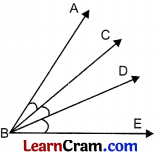# DAV Class 4 Maths Chapter 10 Brain Teasers Solutions

The DAV Maths Book Class 4 Solutions and DAV Class 4 Maths Chapter 10 Brain Teasers Solutions of Angles offer comprehensive answers to textbook questions.

## DAV Class 4 Maths Ch 10 Brain Teasers Solutions

Question 1.
(a) The angle made between the hands of a clock at 2 O’ clock is a ___________ angle.
(i) Obtuse
(ii) Acute
(iii) Right
(iv) Straight
(ii) Acute

(b) There are ___________ right angles in a straight angle.
(i) 3
(ii) 2
(iii) 1
(iv) 4
(ii) 2

(c) The two rays forming an angle are called the ___________ of the angle.
(i) Arms
(ii) Angle
(iii) Degree
(iv) Vertex
(i) Arms

(d) An angle of measure equal to ___________ is an obtuse angle.
(i) 89°
(ii) 91°
(iii) 80°
(iv) 81°
(ii) 91°

(e) Sum of four angles of a rectangle is equal to degrees.
(i) 180°
(ii) 90°
(iii) 360°
(iv) 100°
(iii) 360°Question 2.
Fill in the blanks.
(a) An angle has ___________ vertex and ___________ arms.
One, two

(b) A 75° angle is an ___________ angle.
Acute

(c) Each angle of ___________ a rectangle is of degrees.
90°

(d) There are ___________ angles in a square.
4

(e) There are ___________ angles in a triangle.
3

(f) An angle equal to 180° is called a ___________ angle.
Straight.

Question 3.
Draw the following angles of any measure.
(a) ∠AOB
Let us draw ∠AOB = 55°(b) ∠XYZ
Let us draw ∠XYZ = 90°(c) ∠MNP
Let us draw ∠MNP = 95°Question 4.
How many angles are there in the following figures?5 angles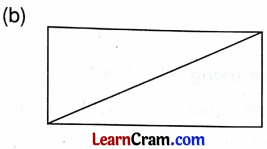8 angles

Question 5.
Draw a line segment PQ of length 6 cm. At P draw an angle of measure 60°.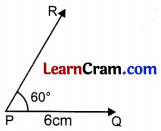Question 6.
Construct the following angles.
(a) 110°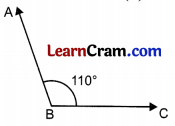(b) 90°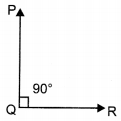(c) 25°(d) 165°Question 7.
Look at the given figure and answer the following.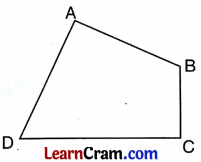(a) How many acute angles are there?
1

(b) How many obtuse angles are there?
2

(c) How many right angles are there?
1

(d) Is ∠ABC an acute angle?
No

(e) ∠DCB = ___________ degree.
90Question 8.
Look at the letter and complete the table.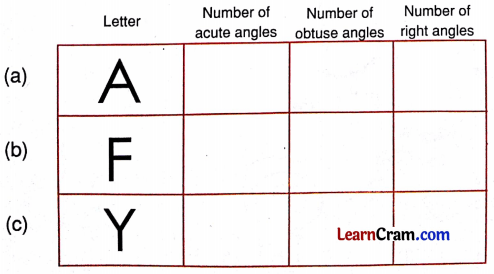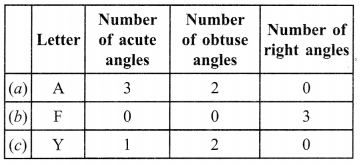Question 9.
The hour hand and minute hand of a clock are making an angle of 90° with each other. The time is ___________ O’ clock or ___________ O’ clock.
3 O’ clock or 9 O’ clock

Question 10.
Name all the angles in the given figure.∠AOB, ∠BOC, ∠AOC

Question 11.
Compare using ‘>’, ‘<‘ or ‘=’.
(a) Acute angle __ Obtuse angle
(b) Right angle __ Obtuse angle
(c) Straight angle __ Two right angles
(a) <
(b) <
(c) =

Question 1.
Complete the table:Angle Arm ∠Q $$\overrightarrow{\mathrm{PQ}}, \overrightarrow{\mathrm{QR}}$$ ∠Y $$\overrightarrow{\mathrm{XY}}, \overrightarrow{\mathrm{YZ}}$$Question 2.
Draw the following angles:
(a) Acute angle ∠MNO.
(b) Obtuse angle ∠PQR.
(c) Right angle ∠LMN.
(d) Straight angle ∠XYZ.
(a) Acute angle can be drawn between 0° and 90°.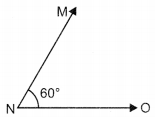∠MNO = 60°

(b) Obtuse angle must be greater than 90° or lie between 90° and 180°.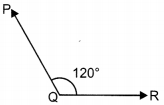∠PQR = 120°

(c) Right angle∠LMN = 90°

(d) Straight angle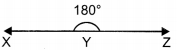∠XYZ = 90°

Question 3.
Identify the following angles:(i) Right angle
(ii) Obtuse angle
(iii) Zero angle
(iv) Acute angle
(v) Acute angle

Question 4.
Put ‘<‘, ‘>’ or ‘=’ sign.
(i) Obtuse angle ( ) Acute angle
(ii) Right angle ( ) Two right angles
(iii) Right angle ( ) Acute angle
(iv) Obtuse angle ( ) Zero angle
(v) Straight angle ( ) Zero angle